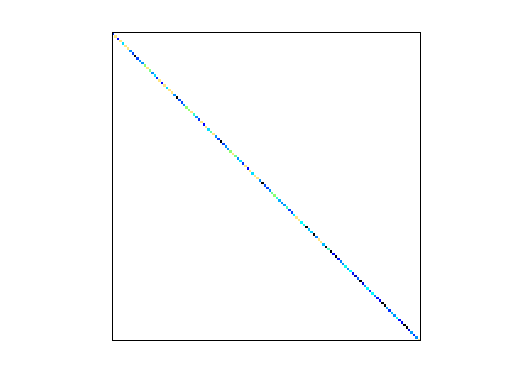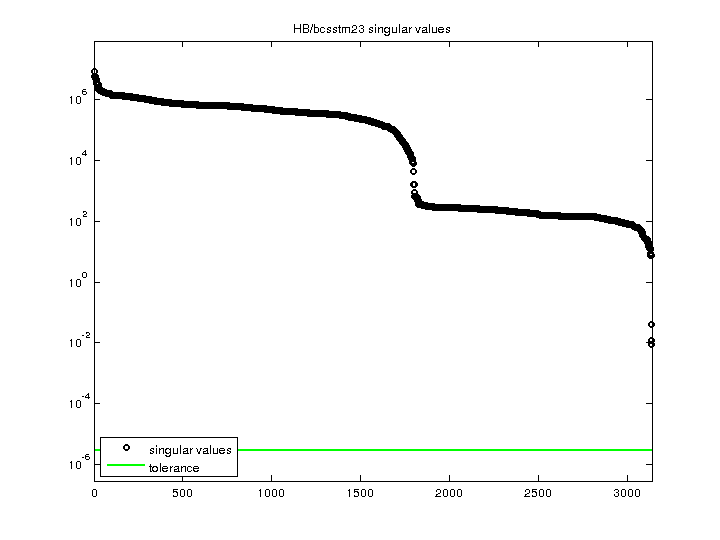Matrix: HB/bcsstm23

Description: SYMMETRIC MASS MATRIX - PORTION OF A 3D GLOBALLY TRIANGLULAR BLDG(undirected graph drawing)• Matrix group: HB
• download as a MATLAB mat-file, file size: 24 KB. Use UFget(73) or UFget('HB/bcsstm23') in MATLAB.

 Matrix properties number of rows 3,134 number of columns 3,134 nonzeros 3,134 structural full rank? yes structural rank 3,134 # of blocks from dmperm 3,134 # strongly connected comp. 3,134 explicit zero entries 0 nonzero pattern symmetry symmetric numeric value symmetry symmetric type real structure symmetric Cholesky candidate? yes positive definite? yes

 author J. Lewis editor I. Duff, R. Grimes, J. Lewis date 1984 kind structural problem 2D/3D problem? yes

 Ordering statistics: result nnz(chol(P*(A+A'+s*I)*P')) with AMD 3,134 Cholesky flop count 3.1e+03 nnz(L+U), no partial pivoting, with AMD 3,134 nnz(V) for QR, upper bound nnz(L) for LU, with COLAMD 3,134 nnz(R) for QR, upper bound nnz(U) for LU, with COLAMD 3,134

 SVD-based statistics: norm(A) 8.27035e+06 min(svd(A)) 0.00874194 cond(A) 9.46054e+08 rank(A) 3,134 sprank(A)-rank(A) 0 null space dimension 0 full numerical rank? yes

 singular values (MAT file): click here SVD method used: s = svd (full (A)) ; status: ok